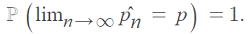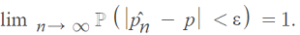# Law of Large Numbers / Law of Averages

Share on

Statistics Definitions > Law of Large Numbers & The Law of Averages

Contents:

## What is the Law of Large Numbers?

The Law of Large Numbers shows us that if you take an unpredictable experiment and repeat it enough times, what you’ll end up with is an average.

Let’s say you had an experiment where you were tossing a fair coin with probability p (for a fair coin, p = 0.5). For a few coin tosses, you might not come anywhere near p = 0.5. However, as you perform more and more experiments, your experimental proportion of outcomes with probability p̂n will converge to p.

In simple terms: If you repeated an experiment many, many, many times…you’ll start to see a, expected pattern, which make it easier to figure out probabilities.A simple example: throw a die and you’ll get a random number (for a six-sided die, you’ll get 1,2,3,4,5,6). Throw the die 100,000 times and you’ll get an average of 3.5 — which is the expected value.

## Law of Large Numbers vs Central Limit Theorem

Both laws tell us that given a sufficiently large amount of data points, those data points will result in predictable behaviors. The Central Limit Theorem shows that as a sample size tends to infinity, the shape of the sample distribution will approach the normal distribution; the Law of Large Numbers shows you where the center of that normal curve is likely to be located.

## Strong and Weak Law of Large Numbers

The Law of Large numbers is sometimes called the Weak Law of Large Numbers to distinguish it from the Strong Law of Large Numbers. The two versions of the law are different depending on the mode of convergence. As the name suggests, the weak law is weaker than the strong law. Basically, the weak law is where the sample mean converges to the expected mean in mean square and in probability; the strong law of large numbers is where the sample mean Mn converges to the expected mean μ with probability 1.

The strong law implies the weak law, but it considers infinite sequence of outcomes. Therefore, the weak law is often better for practical applications.

The strong law of numbers can be defined more precisely with a few terms from calculus:

Given an infinite sequence of observations:Where:

• ℙ = probability of an event,
• p̂ = experimental proportion,
• p = expected proportion,
• lim = limit.

This is stating that, with a probability of 1, the limit
of the sequence p̂ will equal p.

The weak law of large numbers states that as n → ∞, the probability that the inequality |p̂np| ≥ ε goes to zero, regardless of how small ε is. In notation, that’s:(Where epsilon (ε) is a tiny number, close to zero).

The following proof uses the epsilon-delta definition of a limit to show that the strong LLN implies the weak LLN (Kjos-Hanssen, 2019):

Let ε > 0 be given. Sincewe have:
ℙ(there is an m such that for all n ≥ m, |p̂n – p| < ε) = 1.
We can conclude that, for each δ > 0, there is an m such that ℙ(for all n ≥ m, |p̂n – p| < ε) ≥ 1 – δ. In particular, for all n ≥ m, ℙ(|p̂n – p| < ε) ≥ 1 – δ.

## Law of Averages

The law of averages sometimes sneaks into textbooks in place of the Law of Large Numbers. The two terms are not, technically, interchangeable.

• The Law of Large Numbers: If you take an unpredictable experiment and repeat it enough times (i.e. for a very big number), what you’ll end up with is an average.
• The Law of Averages: the belief that the Law of Large Numbers also applies to small numbers as well.

They’re basically the same thing, except that the law of averages stretches the law of large numbers to apply for small numbers as well. The law of large numbers is a statistical concept that always works; the law of averages is a layperson’s term that sometimes works…and sometimes doesn’t.

## The Law of Averages and Why it Doesn’t Work

A common misconception is that lottery numbers that haven’t appeared in a while are due to come up. They aren’t; any one number is just as likely as the next to come up. Think about it: if there was any pattern at all behind the lottery numbers (there isn’t), all us mathematicians would be rich. And most of us are still broke.

One famous example of the law of averages in gambling is called the Gambler’s Fallacy (see below), which is believing a losing streak will “even out” in the end. It won’t. For example, let’s say you’re betting on red on a roulette table, but you lose ten times in a row with every ball landing on black. You think that the law of averages will result in a streak of reds. It wont. The probability of getting a red on any spin is 1/2, no matter how many times it’s spun. A losing streak is just that: a losing streak. The next spin is just as likely to be black as it is red.

The reason that the reds and blacks won’t even out is because you have a small amount of spins. You could stay at the wheel for a couple of hours and bet 200 times, but that is still a relatively small number (compared to a million or a trillion) and the law of averages says nothing about small numbers. If you stayed at the wheel for an infinite amount of spins (in practice, a couple of million should do it), eventually the spins will even out to 50% black and 50% red.If you still aren’t convinced, try this Wolfram dice rolling calculator. You can set the number of rolls as a small amount (say, up to 50) and you’ll see some pretty random results. Up the number of rolls to a few thousand and you’ll see the results start to converge to an average.

## What is the Gambler’s Fallacy?

The gambler’s fallacy is the mistaken belief that if you get a lucky streak, the odds are that in the long run your chances will even out. It also works in reverse with an unlucky streak. A simple example: you toss a coin 10 times, hoping to get heads. Logic tells you that if you toss the coin 10 times, 5 tosses will result in tails and 5 will result in heads. The first five coin tosses are heads. You (mistakenly) think that the next five tosses will probably be five tails.Q. You toss a fair coin 10 times in a row and get 10 heads. If you continue to toss the coin until you’ve tossed it a hundred times, will the heads and tails even out so you end up with roughly 50 tails and 50 heads?

If you answered yes, then you’ve just fallen into the gambler’s fallacy. If you toss a coin 10 times and get all heads, that uncommon steak isn’t going to be evened out by a streak of tails in the future.

A fallacy is a mistaken belief, usually a belief based on a faulty argument. The reasoning (or argument) in answering “yes” to the above question is that given a fair coin, if you toss it enough times, you’ll come up with 50 percent heads and 50 percent tails.* And usually tossing it a hundred times is enough to even out the heads/tails. However, a streak of heads (or tails) is very unusual. In fact, you have the tiny probability of 00.098% of that happening. Now consider what happens on the 13th coin toss: you have a 50 percent chance of a heads or a tails. And so for the 14th toss, and the 15th toss. So you’re likely to end up with (for the next 90 tosses) 45 heads and 45 tails, giving you a total of 55 heads and 45 tails.

A second example of the gambler’s fallacy; you play an online game with one of your friends and over the last year you’ve won 50% of your games. Your friend has a winning streak of 4 games in a row. You mistakenly think that the next 4 games will probably be wins for you as you are “due” for a win.

## The Inverse Gambler’s Fallacy

The reversal of the Gambler’s fallacy is also a fallacy, where (because the gambler thinks they are on a “lucky streak”) that they are more likely to get the same result (in the first example above, more tails).

Gambler’s fallacy is also known as the Monte Carlo Fallacy or the fallacy of maturity of chances.

A joke (retold on Penn State’s site) tells the tale of the man stopped from boarding an airplane when he was found to be carrying a bomb. When questioned as to why he was taking a bomb, he reasons, “The chances of an airplane having a bomb on it are very small, and certainly the chances of having two are almost none!” Similarly, the hero in The World According to Garp buys a house that has just had a plane crash into it. His reasoning is that the chances of another plane crashing into it are practically zero.

Or how about holding a lightning rod in a storm. You’re chances of getting hit by lightning are pretty high. If you get hit once (and survive), are you going to keep on holding the lightning rod? Probably not&hellip’

*Technically speaking, as long as the outcomes are independent events, the probability is exactly 50 percent.

## References

Kjos-Hanssen, B. (2019). Statistics for Calculus Students. Retrieved April 28, 2021 from: https://dspace.lib.hawaii.edu/bitstream/10790/4572/s4cs.pdf

CITE THIS AS:
Stephanie Glen. "Law of Large Numbers / Law of Averages" From StatisticsHowTo.com: Elementary Statistics for the rest of us! https://www.statisticshowto.com/law-large-numbers/
---------------------------------------------------------------------------Need help with a homework or test question? With Chegg Study, you can get step-by-step solutions to your questions from an expert in the field. Your first 30 minutes with a Chegg tutor is free!